Courses

# NEET Previous Year Questions (2014-20): Electromagnetic Waves Class 12 Notes | EduRev

## NEET : NEET Previous Year Questions (2014-20): Electromagnetic Waves Class 12 Notes | EduRev

The document NEET Previous Year Questions (2014-20): Electromagnetic Waves Class 12 Notes | EduRev is a part of the NEET Course Physics 31 Years NEET Chapterwise Solved Papers.
All you need of NEET at this link: NEET

Q.1. The ratio of contributions made by the electric field and magnetic field components to the intensity of an electromagnetic wave is : (c = speed of electromagnetic waves)     (2020)
A: 1 : c
B: 1 : c2
C: c : 1
D: 1 : 1

Ans: D
Energy distribution is same so ration of electric field and magnetic field will be 1 : 1

Q.2. Which colour of the light has the longest wavelength?    (2019)
A: Red
B: Blue
C: Green
D: Violet
Ans: A
Solution:

Red has the longest wavelength among the given options.

Q.3. An em wave is propagating in a medium with a velocity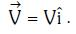The instantaneous oscillating electric field of this em wave is along +y axis. Then the direction of oscillating magnetic field of the em wave will be along :-    (2018)
A: –z direction
B: +z direction
C: –y direction
D: –x direction
Ans:
B
Solution: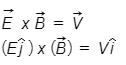(Therefore, electric field vector is along + y axis)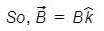i.e., direction of magnetic filed vector is along + z direction.

Q.4. In an electromagnetic wave in free space the root mean square value of the electric field is Erms = 6V/m. The peak value of the magnetic field is :-    (2017)
A: 2.83 × 10–8 T
B: 0.70 × 10–8 T
C: 4.23 × 10–8 T
D: 1.41 × 10–8 T
Ans: A
Solution: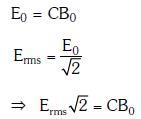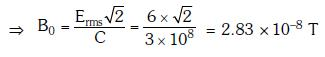Q.5. Out of the following options which one can be used to produce a propagating electromagnetic wave ?    (2016)
A: An accelerating charge
B: A charge moving at constant velocity
C: A stationary charge
D: A charge less particle
Ans:
A
Solution:

An electric charge at rest has electric field in the region around it, but magnetic field is absent. A moving charge produces both electric and magnetic field. When a charge is moving with constant velocity, electric and magnetic field will not change with time. Therefore, no electromagnetic wave will be produced.
But, if the charge is moving with non-zero acceleration, electric and magnetic field will change with space and time. Electromagnetic waves will be produced.
Therefore, accelerated charge emits electromagnetic waves.

Offer running on EduRev: Apply code STAYHOME200 to get INR 200 off on our premium plan EduRev Infinity!

28 docs|50 tests

,

,

,

,

,

,

,

,

,

,

,

,

,

,

,

,

,

,

,

,

,

;